Open in App
Not now

# How to insert Strings into an AVL Tree

• Difficulty Level : Hard
• Last Updated : 11 Oct, 2021

AVL Tree is a self-balancing Binary Search Tree (BST) where the difference between heights of left and right subtrees cannot be more than one for all nodes.

Examples: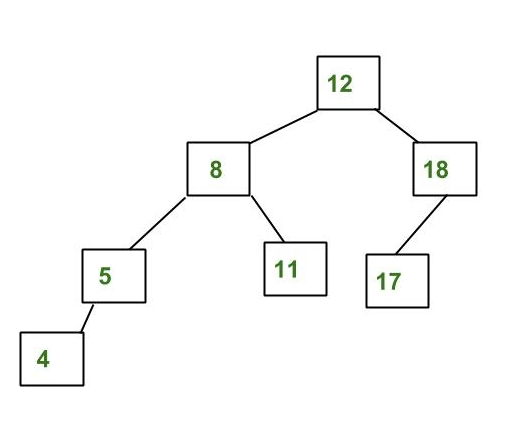The above tree is AVL because the differences between the heights of the left and right subtrees for every node are less than or equal to 1. Below is the example that is not an AVL Tree: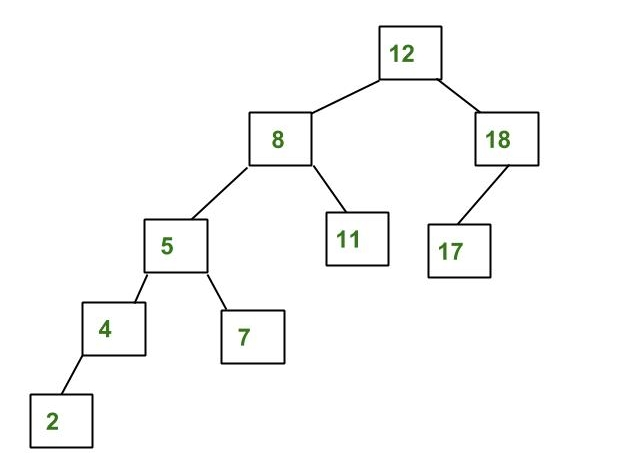The above tree is not AVL because the differences between the heights of the left and right subtrees for 8 and 12 are greater than 1. It can be defined as a type of binary search tree that has all its nodes with a balance factor of -1, 0, or 1.

What is the balance factor: It is the difference in height between the two subtrees. (Balance Factor: Height of Left subtree – Height of right subtree)

### Insertion of Strings in AVL Tree:

Below example demonstration of inserting the days of the week in the order: {Tuesday, Monday, Thursday, Saturday, Sunday, Friday, Wednesday}

Approach:

• If the node is empty(NULL), create the node with the first value given.
• If the new node is less than the previous node then insert the new node at the left
• If the new node is greater than the previous node then insert the new node at the right

Check if the tree is balanced:

• If the balance factor is greater than 1 then it can be either:
• Left-Left Case (then a single rotation is required to make it balanced).
• Or Left-Right Case(Double rotation required).
• If the balance factor is lesser than -1 then it can be either:
• Right-Right Case (Single rotation required).
• Or Right-Left Case (Double rotation required)

This process should be repeated for the insertion of all of the remaining nodes.

Points to Remember:

• Insert nodes accordingly: To make sure that the given tree remains AVL after every insertion, augment the standard BST insert operation to perform some re-balancing. Following are two basic operations that can be performed to re-balance a BST without violating the BST property (keys(left) < key(root) < keys(right)).
• Check the Balance Factor: The balance factor should always be -1, 0, 1 if not then there is a need to rotate the tree accordingly.

Steps To Create AVL Tree:

• So according to the given order, let’s insert Tuesday, as it has no node so it has a balance factor of 0.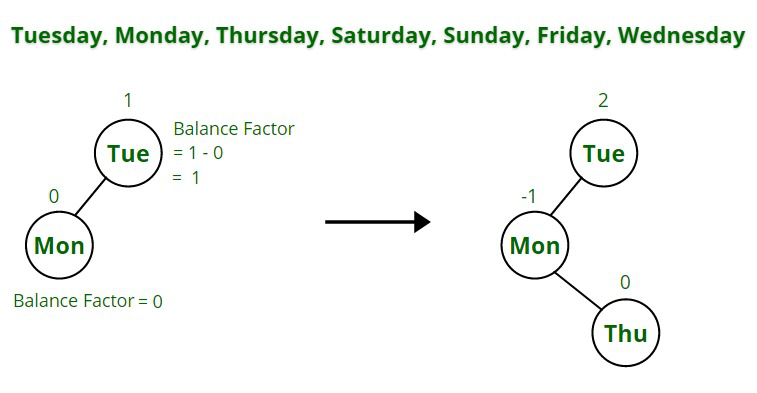• The next step is to insert Monday to the left of Tuesday as it is alphabetically smaller(M < T). There is a need to check the balance factor of each node before inserting a new node. The tree is balanced as Tuesday is balanced because it has one subtree towards the left:

Balance Factor = height of the left subtree – the height of right subtree = 1 – 0 = 1

Therefore, the tree is balanced. Monday is also balanced because of balance factor 0.

• Next, insert Thursday. It is inserted to the left of Tuesday (as Th < Tu) and it is inserted to the right of Monday (as T>M). Now if the balance factor, is checked it can be seen that the balance factor of Tuesday is 2 so it is unbalanced, so there is a need to rotate the tree to make it balanced.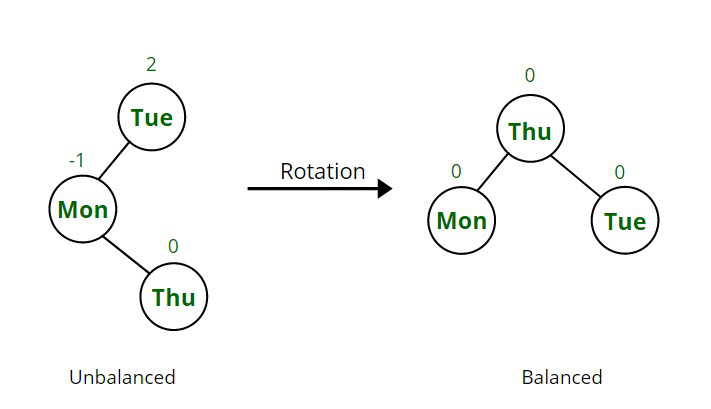Note: In case if we don’t remember the rules of rotation of AVL tree, still it can be balanced we just need to remember that: Left node < Root < Right node.

Example:

5                        4
/                       /   \
3     —–>      3       5
\
4

• After that, insert Saturday, to the left of Thursday (S < T) and to the right of Monday (M < S).
• Sunday is inserted to the right of Saturday (Sa < Su). Now the tree is unbalanced, so it is rotated to make it again balanced.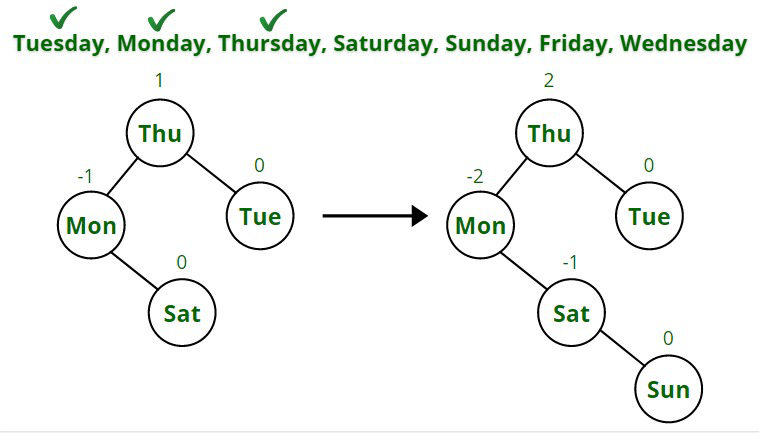• Friday is inserted following the same rules and the balance factor of Thursday becomes 2, so rotation is again done.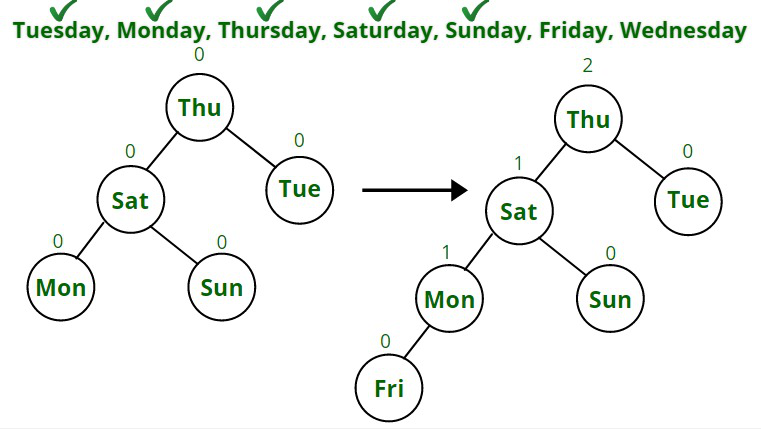• After rotation, now the last node Wednesday is inserted to the right of Tuesday (as T<W).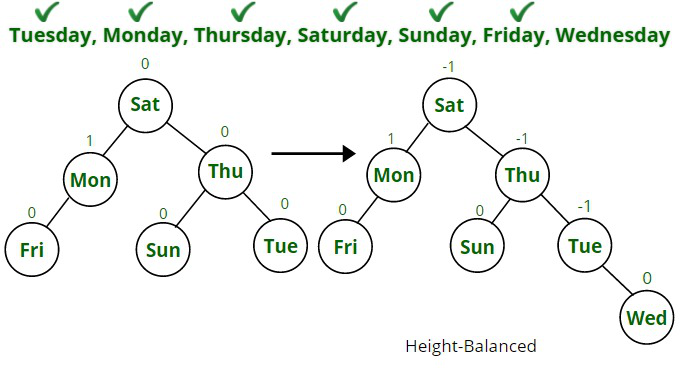If the AVL tree is checked, now the entire AVL tree is height-balanced as all the nodes have balance factors -1, 0, 1.

My Personal Notes arrow_drop_up